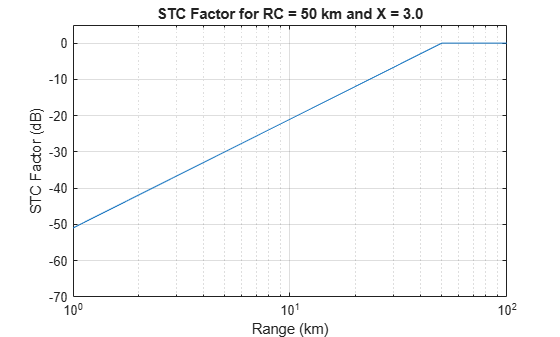# stcfactor

Sensitivity time control (STC) factor

## Syntax

``FSTC = stcfactor(R,RC,X)``

## Description

example

````FSTC = stcfactor(R,RC,X)` computes the range-dependent STC factor, FSTC, in dB given , `R`, the STC cutoff range, `RC`, and an exponent ,`X`.```

## Examples

collapse all

Compute the STC factor for the STC cutoff range of `50` km and the STC exponent of `3.0`.

```R = 0:1e3:100e3; RC = 50e3; X = 3.0; FSTC = stcfactor(R,RC,X);```

Plot the STC factor.

```semilogx(R*1e-3,FSTC) grid on; xlabel('Range (km)'); ylabel('STC Factor (dB)'); ylim([-70 5]); title('STC Factor for RC = 50 km and X = 3.0');```## Input Arguments

collapse all

Range at which to compute `FSTC`, specified as a positive scalar or length-J vector in meters.

STC cutoff range, specified as a positive scalar or as a length-`K` vector in meters.

Exponent to maintain the target detectability, specified as a positive scalar in the range of `[3,4]` or as a length-K vector with each element in the range of `[3,4]`. The exponent maintains the target detectability below the STC cutoff range.

## Output Arguments

collapse all

Range-dependent STC factor, returned as a J-by-K matrix in dB with rows corresponding to the values in `R` and columns corresponding to the ranges in `RC` and `X`.

## Extended Capabilities

### C/C++ Code GenerationGenerate C and C++ code using MATLAB® Coder™.